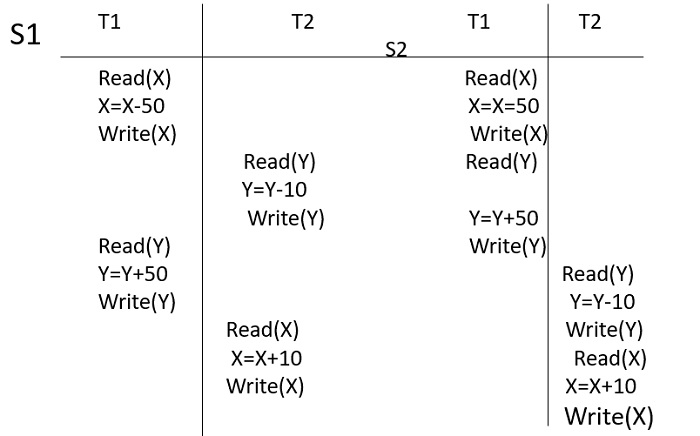# When do we say the schedule is conflict equivalent(DBMS)?

Two schedules are said to be conflict equivalent if the order of any two conflicting operations are the same in both the schedules.

Also, a concurrent schedule S is conflict equivalent to a serial schedule S’, if we can obtain S’ out of S by swapping the order of execution of non-conflicting instructions.

## Example 1Even if the schedule S1 keeps the database in the consistent state, we cannot convert it into a serial schedule and hence we conclude that the schedule is not conflict equivalent to any of the serial schedules.

So, instead of considering only the read and write operation, we will also consider the intermediate operations which will result in a new form of serializability known as view serializability.

So by seeing above transactions S1 is the conflict equivalent of S2.

## Example 2

Two schedules are said to be conflict equivalent if the order of any two conflicting operations are the same in both the schedules.

The pre-requisite conditions for conflicting operations are −

• The two conflicting operations should belong to two different transactions.

• They should be acting over the same database or variable say x.

• At least one of the operations should be "Write". For example, operations should be like Read-write; Write-Write; Write-Read

Schedule 1Schedule 2
R1(x)R1(y)
W2(x)R1(x)
R1(y)W2(x)

Here in the example,

• R(x) - Read operation over variable x

• W(x) - Write operation over variable x

• R1W2 is the conflicting operation. As the order is the same in both the schedules.